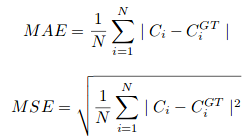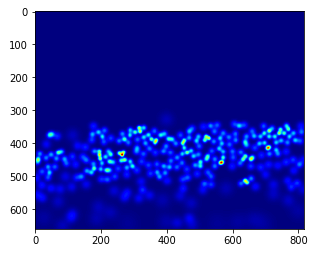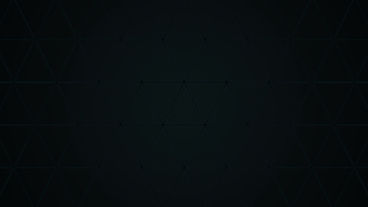Search
•Analytikus

# It’s a Record-Breaking Crowd! A Must-Read Tutorial to Build your First Crowd Counting Model using DL

Artificial Intelligence and Machine Learning is going to be our biggest helper in coming decade!

Today morning, I was reading an article which reported that an AI system won against 20 lawyers and the lawyers were actually happy that AI can take care of repetitive part of their roles and help them work on complex topics. These lawyers were happy that AI will enable them to have more fulfilling roles.

Today, I will be sharing a similar example – How to count number of people in crowd using Deep Learning and Computer Vision? But, before we do that – let us develop a sense of how easy the life is for a Crowd Counting Scientist.

### Act like a Crowd Counting Scientist

Let’s start!

Can you help me count / estimate number of people in this picture attending this event?Source: ShanghaiTech Dataset

You get the hang of it. By end of this tutorial, we will create an algorithm for Crowd Counting with an amazing accuracy (compared to humans like you and me). Will you use such an assistant?

P.S. This article assumes that you have a basic knowledge of how convolutional neural networks (CNNs) work. You can refer to the below post to learn about this topic before you proceed further:

A Comprehensive Tutorial to learn Convolutional Neural Networks from Scratch

## What is Crowd Counting?

Crowd Counting is a technique to count or estimate the number of people in an image. Take a moment to analyze the below image:Source: ShanghaiTech Dataset

Can you give me an approximate number of how many people are in the frame? Yes, including the ones present way in the background. The most direct method is to manually count each person but does that make practical sense? It’s nearly impossible when the crowd is this big!

Crowd scientists (yes, that’s a real job title!) count the number of people in certain parts of an image and then extrapolate to come up with an estimate. More commonly, we have had to rely on crude metrics to estimate this number for decades.

Surely there must be a better, more exact approach?

Yes, there is!

While we don’t yet have algorithms that can give us the EXACT number, most computer vision techniques can produce impressively precise estimates. Let’s first understand why crowd counting is important before diving into the algorithm behind it.

## Why is Crowd Counting useful?

Let’s understand the usefulness of crowd counting using an example. Picture this – your company just finished hosting a huge data science conference. Plenty of different sessions took place during the event.

You are asked to analyze and estimate the number of people who attended each session. This will help your team understand what kind of sessions attracted the biggest crowds (and which ones failed in that regard). This will shape next year’s conference, so it’s an important task!There were hundreds of people at the event – counting them manually will take days! That’s where your data scientist skills kick in. You managed to get photos of the crowd from each session and build a computer vision model to do the rest!

There are plenty of other scenarios where crowd counting algorithms are changing the way industries work:

Counting the number of people attending a sporting eventEstimating how many people attended an inauguration or a march (political rallies, perhaps)Monitoring of high-traffic areasHelping with staffing allocation and resource allotment

Can you come up with some other use cases? Let me know in the comments section below! We can connect and try to figure out how we can use crowd counting techniques in your scenario.

## Understanding the Different Computer Vision Techniques for Crowd Counting

Broadly speaking, there are currently four methods we can use for counting the number of people in a crowd:

### 1. Detection-based methods

Here, we use a moving window-like detector to identify people in an image and count how many there are. The methods used for detection require well trained classifiers that can extract low-level features. Although these methods work well for detecting faces, they do not perform well on crowded images as most of the target objects are not clearly visible.

### 2. Regression-based methods

We were unable to extract low level features using the above approach. Regression-based methods come up trumps here. We first crop patches from the image and then, for each patch, extract the low level features.

### 3. Density estimation-based methods

We first create a density map for the objects. Then, the algorithm learn a linear mapping between the extracted features and their object density maps. We can also use random forest regression to learn non-linear mapping.

### 4. CNN-based methods

Ah, good old reliable convolutional neural networks (CNNs). Instead of looking at the patches of an image, we build an end-to-end regression method using CNNs. This takes the entire image as input and directly generates the crowd count. CNNs work really well with regression or classification tasks, and they have also proved their worth in generating density maps.

CSRNet, a technique we will implement in this article, deploys a deeper CNN for capturing high-level features and generating high-quality density maps without expanding the network complexity. Let’s understand what CSRNet is before jumping to the coding section.

## Understanding the Architecture and Training Method of CSRNet

CSRNet uses VGG-16 as the front end because of its strong transfer learning ability. The output size from VGG is ⅛th of the original input size. CSRNet also uses dilated convolutional layers in the back end.

But what in the world are dilated convolutions? It’s a fair question to ask. Consider the below image:The basic concept of using dilated convolutions is to enlarge the kernel without increasing the parameters. So, if the dilation rate is 1, we take the kernel and convolve it on the entire image. Whereas, if we increase the dilation rate to 2, the kernel extends as shown in the above image (follow the labels below each image). It can be an alternative to pooling layers.

### Underlying Mathematics (Recommended, but optional)

I’m going to take a moment to explain how the mathematics work. Note that this isn’t mandatory to implement the algorithm in Python, but I highly recommend learning the underlying idea. This will come in handy when you need to tweak or modify your model.

Suppose we have an input x(m,n), a filter w(i,j), and the dilation rate r. The output y(m,n) will be:We can generalize this equation using a (k*k) kernel with a dilation rate r. The kernel enlarges to:

([k + (k-1)*(r-1)] * [k + (k-1)*(r-1)])

So the ground truth has been generated for each image. Each person’s head in a given image is blurred using a Gaussian kernel. All the images are cropped into 9 patches, and the size of each patch is ¼th of the original size of the image. With me so far?

The first 4 patches are divided into 4 quarters and the other 5 patches are randomly cropped. Finally, the mirror of each patch is taken to double the training set.

That, in a nutshell, are the architecture details behind CSRNet. Next, we’ll look at its training details, including the evaluation metric used.

Stochastic Gradient Descent is used to train the CSRNet as an end-to-end structure. During training, the fixed learning rate is set to 1e-6. The loss function is taken to be the Euclidean distance in order to measure the difference between the ground truth and estimated density map. This is represented as:where N is the size of the training batch. The evaluation metric used in CSRNet is MAE and MSE, i.e., Mean Absolute Error and Mean Square Error. These are given by:Here, Ci is the estimated count:L and W are the width of the predicted density map.

Our model will first predict the density map for a given image. The pixel value will be 0 if no person is present. A certain pre-defined value will be assigned if that pixel corresponds to a person. So, calculating the total pixel values corresponding to a person will give us the count of people in that image. Awesome, right?

And now, ladies and gentlemen, it’s time to finally build our own crowd counting model!

## Building your own Crowd Counting model

We will implement CSRNet on the ShanghaiTech dataset. This contains 1198 annotated images of a combined total of 330,165 people. You can download the dataset from here.

Use the below code block to clone the CSRNet-pytorch repository. This holds the entire code for creating the dataset, training the model and validating the results:

`git clone https://github.com/leeyeehoo/CSRNet-pytorch.git`

Please install CUDA and PyTorch before you proceed further. These are the backbone behind the code we’ll be using below.

Now, move the dataset into the repository you cloned above and unzip it. We’ll then need to create the ground truth values. The make_dataset.ipynb file is our savior. We just need to make minor changes in that notebook:

# importing librariesimport h5pyimport scipy.io as ioimport PIL.Image as Imageimport numpy as npimport osimport globfrom matplotlib import pyplot as pltfrom scipy.ndimage.filters import gaussian_filterimport scipyimport jsonfrom matplotlib import cm as CMfrom image import *from model import CSRNetimport torchfrom tqdm import tqdm%matplotlib inlineview rawimport_libraries.py hosted with ❤ by GitHub

# function to create density maps for imagesdef gaussian_filter_density(gt): print (gt.shape) density = np.zeros(gt.shape, dtype=np.float32) gt_count = np.count_nonzero(gt) if gt_count == 0: return density pts = np.array(list(zip(np.nonzero(gt), np.nonzero(gt)))) leafsize = 2048 # build kdtree tree = scipy.spatial.KDTree(pts.copy(), leafsize=leafsize) # query kdtree distances, locations = tree.query(pts, k=4) print ('generate density...') for i, pt in enumerate(pts): pt2d = np.zeros(gt.shape, dtype=np.float32) pt2d[pt,pt] = 1. if gt_count > 1: sigma = (distances[i]+distances[i]+distances[i])*0.1 else: sigma = np.average(np.array(gt.shape))/2./2. #case: 1 point density += scipy.ndimage.filters.gaussian_filter(pt2d, sigma, mode='constant') print ('done.') return densityview rawdensity_map.py hosted with ❤ by GitHub

```#setting the root to the Shanghai dataset you have downloaded
# change the root path as per your location of dataset
root = '/home/pulkit/CSRNet-pytorch/'```

Now, let’s generate the ground truth values for images in part_A and part_B:

part_A_train = os.path.join(root,'part_A/train_data','images')part_A_test = os.path.join(root,'part_A/test_data','images')part_B_train = os.path.join(root,'part_B/train_data','images')part_B_test = os.path.join(root,'part_B/test_data','images')path_sets = [part_A_train,part_A_test]view rawground_truth.py hosted with ❤ by GitHub

img_paths = []for path in path_sets: for img_path in glob.glob(os.path.join(path, '*.jpg')): img_paths.append(img_path)view rawimage_location.py hosted with ❤ by GitHubfor img_path in img_paths: print (img_path) mat = io.loadmat(img_path.replace('.jpg','.mat').replace('images','ground-truth').replace('IMG_','GT_IMG_')) img= plt.imread(img_path) k = np.zeros((img.shape,img.shape)) gt = mat["image_info"][0,0][0,0] for i in range(0,len(gt)): if int(gt[i])<img.shape and int(gt[i])<img.shape: k[int(gt[i]),int(gt[i])]=1 k = gaussian_filter_density(k) with h5py.File(img_path.replace('.jpg','.h5').replace('images','ground-truth'), 'w') as hf: hf['density'] = kview rawcreate_density_map.py hosted with ❤ by GitHub

Generating the density map for each image is a time taking step. So go brew a cup of coffee while the code runs.

So far, we have generated the ground truth values for images in part_A. We will do the same for the part_B images. But before that, let’s see a sample image and plot its ground truth heatmap:

`plt.imshow(Image.open(img_paths))`Things are getting interesting!

```gt_file = h5py.File(img_paths.replace('.jpg','.h5').replace('images','ground-truth'),'r')
groundtruth = np.asarray(gt_file['density'])
plt.imshow(groundtruth,cmap=CM.jet)```Let’s count how many people are present in this image:

`np.sum(groundtruth)`

270.32568

Similarly, we will generate values for part_B:

path_sets = [part_B_train,part_B_test]img_paths = []for path in path_sets: for img_path in glob.glob(os.path.join(path, '*.jpg')): img_paths.append(img_path) # creating density map for part_b images for img_path in img_paths: print (img_path) mat = io.loadmat(img_path.replace('.jpg','.mat').replace('images','ground-truth').replace('IMG_','GT_IMG_')) img= plt.imread(img_path) k = np.zeros((img.shape,img.shape)) gt = mat["image_info"][0,0][0,0] for i in range(0,len(gt)): if int(gt[i])<img.shape and int(gt[i])<img.shape: k[int(gt[i]),int(gt[i])]=1 k = gaussian_filter_density(k) with h5py.File(img_path.replace('.jpg','.h5').replace('images','ground-truth'), 'w') as hf: hf['density'] = kview rawpart_b_images.py hosted with ❤ by GitHub

Now, we have the images as well as their corresponding ground truth values. Time to train our model!

We will use the .json files available in the cloned directory. We just have to change the location of the images in the json files. To do this, open the .json file and replace the current location with the location where your images are located.

Note that all this code is written in Python 2. Make the following changes if you’re using any other Python version:

In model.py, change xrange in line 18 to rangeChange line 19 in model.py with: list(self.frontend.state_dict().items())[i].data[:] = list(mod.state_dict().items())[i].data[:]In image.py, replace ground_truth with ground-truth

Made the changes? Now, open a new terminal window and type the following commands:

```cd CSRNet-pytorch
python train.py part_A_train.json part_A_val.json 0 0```

Again, sit back because this will take some time. You can reduce the number of epochs in the train.py file to accelerate the process. A cool alternate option is to download the pre-trained weights from here if you don’t feel like waiting.

Finally, let’s check our model’s performance on unseen data. We will use the val.ipynb file to validate the results. Remember to change the path to the pretrained weights and images.

#importing librariesimport h5pyimport scipy.io as ioimport PIL.Image as Imageimport numpy as npimport osimport globfrom matplotlib import pyplot as pltfrom scipy.ndimage.filters import gaussian_filterimport scipyimport jsonimport torchvision.transforms.functional as Ffrom matplotlib import cm as CMfrom image import *from model import CSRNetimport torch%matplotlib inlineview rawimport_library.py hosted with ❤ by GitHubfrom torchvision import datasets, transformstransform=transforms.Compose([ transforms.ToTensor(),transforms.Normalize(mean=[0.485, 0.456, 0.406], std=[0.229, 0.224, 0.225]), ])view rawtransform.py hosted with ❤ by GitHub#defining the location of datasetroot = '/home/pulkit/CSRNet/ShanghaiTech/CSRNet-pytorch/'part_A_train = os.path.join(root,'part_A/train_data','images')part_A_test = os.path.join(root,'part_A/test_data','images')part_B_train = os.path.join(root,'part_B/train_data','images')part_B_test = os.path.join(root,'part_B/test_data','images')path_sets = [part_A_test]view rawlocation.py hosted with ❤ by GitHub

```#defining the image path
img_paths = []
for path in path_sets:
for img_path in glob.glob(os.path.join(path, '*.jpg')):
img_paths.append(img_path)```
`model = CSRNet()`
```#defining the model
model = model.cuda()```
```#loading the trained weights

Check the MAE (Mean Absolute Error) on test images to evaluate our model:

mae = 0for i in tqdm(range(len(img_paths))): img = transform(Image.open(img_paths[i]).convert('RGB')).cuda() gt_file = h5py.File(img_paths[i].replace('.jpg','.h5').replace('images','ground-truth'),'r') groundtruth = np.asarray(gt_file['density']) output = model(img.unsqueeze(0)) mae += abs(output.detach().cpu().sum().numpy()-np.sum(groundtruth))print (mae/len(img_paths))view rawmae.py hosted with ❤ by GitHub

We got an MAE value of 75.69 which is pretty good. Now let’s check the predictions on a single image:

from matplotlib import cm as cimg = transform(Image.open('part_A/test_data/images/IMG_100.jpg').convert('RGB')).cuda() output = model(img.unsqueeze(0))print("Predicted Count : ",int(output.detach().cpu().sum().numpy()))temp = np.asarray(output.detach().cpu().reshape(output.detach().cpu().shape,output.detach().cpu().shape))plt.imshow(temp,cmap = c.jet)plt.show()temp = h5py.File('part_A/test_data/ground-truth/IMG_100.h5', 'r')temp_1 = np.asarray(temp['density'])plt.imshow(temp_1,cmap = c.jet)print("Original Count : ",int(np.sum(temp_1)) + 1)plt.show()print("Original Image")plt.imshow(plt.imread('part_A/test_data/images/IMG_100.jpg'))plt.show()view rawprediction.py hosted with ❤ by GitHubWow, the original count was 382 and our model estimated there were 384 people in the image. That is a very impressive performance!

Congratulations on building your own crowd counting model!

## End Notes

I encourage you to try out this approach on different images and share your results in the comments section below. Crowd counting has so many diverse applications and is already seeing adoption by organizations and government bodies.

It is a useful skill to add to your portfolio. Quite a number of industries will be looking for data scientists who can work with crowd counting algorithms. Learn it, experiment with it, and give yourself the gift of deep learning!

Some opinions expressed in this article may be those of a guest author and not necessarily Analytikus. From: https://www.analyticsvidhya.com/blog/2019/02/building-crowd-counting-model-python/?utm_source=blog&utm_medium=20-popular-machine-learning-and-deep-learning-articles-on-analytics-vidhya-in-2019